Next: Neutron stars Up: Quantum statistics Previous: White-dwarf stars

## The Chandrasekhar limit

One curious feature of white-dwarf stars is that their radius decreases as their mass increases [see Eq. (696)]. It follows, from Eq. (689), that the mean energy of the degenerate electrons inside the star increases strongly as the stellar mass increases: in fact,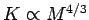. Hence, ifbecomes sufficiently large the electrons become relativistic, and the above analysis needs to be modified. Strictly speaking, the non-relativistic analysis described in the previous section is only valid in the low mass limit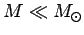. Let us, for the sake of simplicity, consider the ultra-relativistic limit in which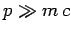.

The total electron energy (including the rest mass energy) can be written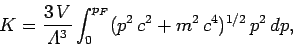(697)

by analogy with Eq. (688). Thus,(698)

giving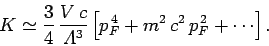(699)

It follows, from the above, that the total energy of an ultra-relativistic white-dwarf star can be written in the form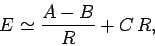(700)

where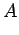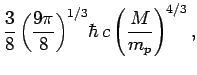(701)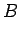(702)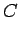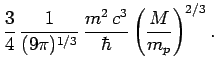(703)

As before, the equilibrium radius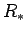is that which minimizes the total energy. However, in the ultra-relativistic case, a non-zero value ofonly exists for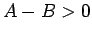. When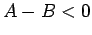the energy decreases monotonically with decreasing stellar radius: in other words, the degeneracy pressure of the electrons is incapable of halting the collapse of the star under gravity. The criterion which must be satisfied for a relativistic white-dwarf star to be maintained against gravity is that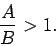(704)

This criterion can be re-written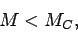(705)

where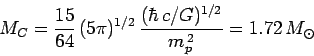(706)

is known as the Chandrasekhar limit, after A. Chandrasekhar who first derived it in 1931. A more realistic calculation, which does not assume constant density, yields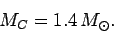(707)

Thus, if the stellar mass exceeds the Chandrasekhar limit then the star in question cannot become a white-dwarf when its nuclear fuel is exhausted, but, instead, must continue to collapse. What is the ultimate fate of such a star?Next: Neutron stars Up: Quantum statistics Previous: White-dwarf stars
Richard Fitzpatrick 2006-02-02